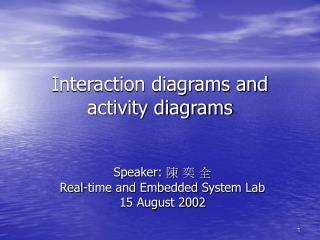DownloadDownload PresentationInteraction diagrams and activity diagrams

# Interaction diagrams and activity diagrams

Télécharger la présentation## Interaction diagrams and activity diagrams

- - - - - - - - - - - - - - - - - - - - - - - - - - - E N D - - - - - - - - - - - - - - - - - - - - - - - - - - -
##### Presentation Transcript

1. Interaction diagrams and activity diagrams Speaker: 陳 奕 全 Real-time and Embedded System Lab 15 August 2002

2. Outline • Introduction of interaction diagrams • Modeling flows of control • Introduction of activity diagrams • Modeling a workflow • Modeling a operation

3. Introduction of interaction diagrams • How do you model system’s dynamic aspects? • To build up storyboards of scenarios. • In UML, you model these storyboards by using interaction diagrams. • storyboard: noun, a series of drawings or pictures that show the outline of the story of a film/movie, etc.

4. Introduction of interaction diagrams (Cont.) • Interaction diagrams contain sequence diagrams and collaboration diagrams. • Sequence diagrams: emphasis the time ordering of messages. • Collaboration diagrams: emphasis the structural organization of objects that send/receive messages. • Both of them are semantically equivalent.

5. Introduction of interaction diagrams (Cont.) • Sequence diagrams • Collaboration diagrams

6. Modeling flows of control • By time ordering: • Set the context for the interaction. • Set the stage for the interaction. • Set the lifeline for each object • Starting with the message • Visualize the nesting of messages (optional) • Specify time or space constraints (optional)

7. Modeling flows of control (Cont.)

8. Modeling flows of control (Cont.) • By organization: • Set the context for the interaction • Set the stage for the interaction • Set the initial properties of each object • Specify the links among • Starting with the messages that initiates this interaction • Specify time or space constraints (optional)

9. Modeling flows of control (Cont.)

10. Introduction of activity diagrams • Consider the workflow associated with building a house. • select site, commission an architect, develop plan, bid plan, construction…until everything is done. • In a real project, there are a lots of parallel activities among various trades. • We can model these dynamic aspects using activity diagrams.

11. Introduction of activity diagrams (Cont.) • Activity diagrams shows the flow from activity to activity. • Activity: an ongoing nonatomic execution within a state machine. Activity Action Made up of executable atomic computations (send a signal, create, destroy)

12. Introduction of activity diagrams (Cont.) • Activity diagrams commonly contain: • Activity states and action states • Transition • Sequential transition, branching, forking and joining.

13. Introduction of activity diagrams (Cont.) • Objects • Swimlane: modeling workflows of business processes. • Flow of object: Usage of dependency relationships and objects

14. Modeling a workflow • To model a workflow • Establish a focus for the workflow. • Select the business objects. • Identify the conditions of the workflow’s states. • Specify the activities and actions and render them in diagrams • Render transitions the connect activity and action states. • Render important objects in the activity diagrams.

15. Modeling a workflow (Cont.)

16. Modeling a operation • To model a operation: • Collect the abstractions that are involved in this operation • Identify the precondition and postcondition • Specify the activities and actions and render them in diagrams • Specify conditional paths and iteration. • Use forking and joining to specify parallel flows of control

17. Modeling a operation Signature: one parameter and one return value